# KSEEB Solutions for Class 8 Maths Chapter 11 Congruence of Triangles Ex 11.1

In this chapter, we provide KSEEB SSLC Class 8 Maths Chapter 11 Congruence of Triangles Ex 11.1 for English medium students, Which will very helpful for every student in their exams. Students can download the latest KSEEB SSLC Class 8 Maths Chapter 11 Congruence of Triangles Ex 11.1 pdf, free KSEEB SSLC Class 8 Maths Chapter 11 Congruence of Triangles Ex 11.1 pdf download. Now you will get step by step solution to each question.

### Karnataka State Syllabus Class 8 Maths Chapter 11 Congruence of Triangles Ex 11.1

Question 1.
1. Identify the corresponding sides and corresponding angles in the following conruent triangles. and PR & XZ corresponding angles.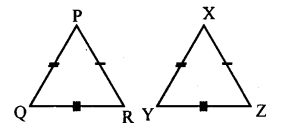Corresponding sides PQ & XY, QR & YZ, and PR & XZ corresponding angles.
∠P & ∠X, ∠Q & ∠Y, and ∠R and ∠Z.

2.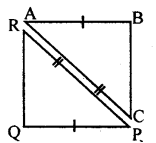Corresponding sides AB & QP, AC & RP and BC & RQ corresponding angles.
∠A & ∠P , ∠B & ∠Q and ∠C &∠R

Question 2.
Pair of the congruent triangle and incomplete statements related to them are given below. Observe the figures carefully and fill up the blanks
a. In the adjoining figure If ∠ L =∠F, then AB = _____ and BC = ______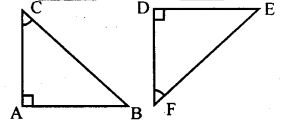AB = DE, BC = EF

b. In the adjoining figure if BC = EF, then ∠C = ______ and ∠A =_______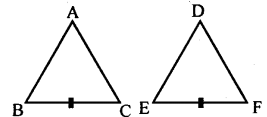∠C = ∠F and ∠A =∠D

c. In the adjoining figure If AC = CE and Δ ABC ≅ ΔDEC then ∠D = ______ and ∠A = _______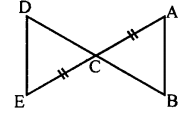∠D = ∠B and ∠A =∠E

All Chapter KSEEB Solutions For Class 8 maths

—————————————————————————–

All Subject KSEEB Solutions For Class 8

*************************************************

I think you got complete solutions for this chapter. If You have any queries regarding this chapter, please comment on the below section our subject teacher will answer you. We tried our best to give complete solutions so you got good marks in your exam.

If these solutions have helped you, you can also share kseebsolutionsfor.com to your friends.

Best of Luck!!